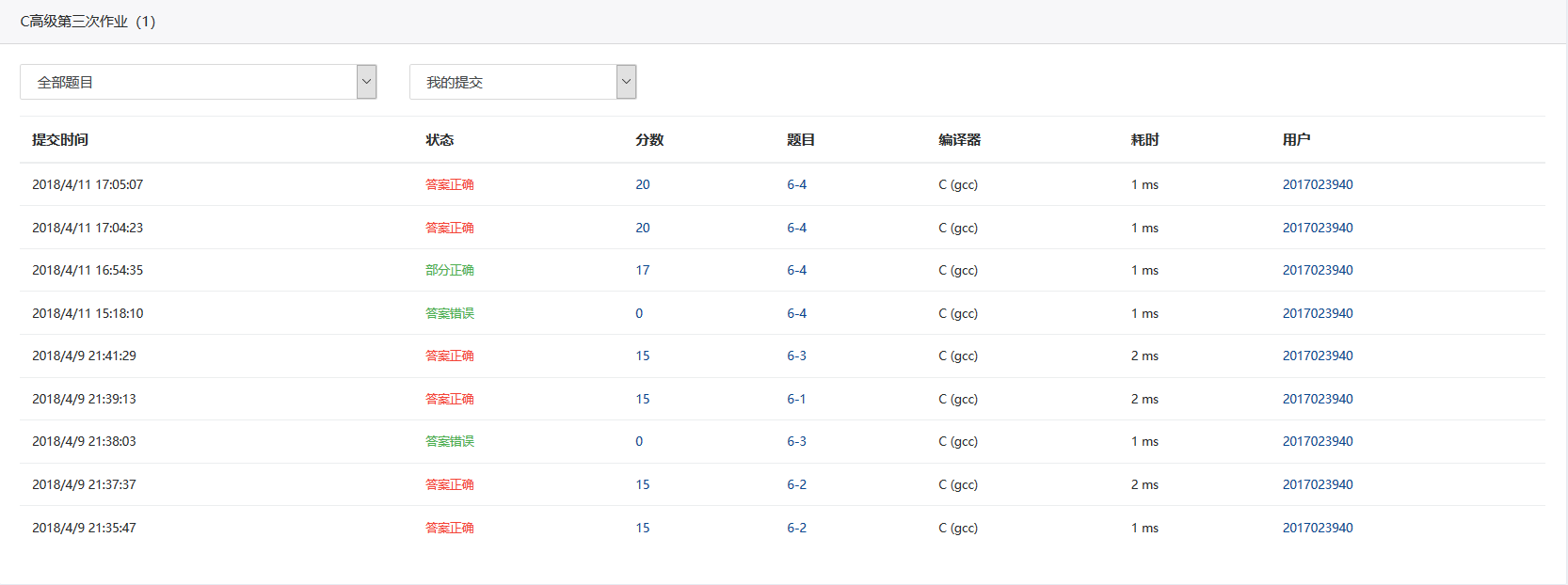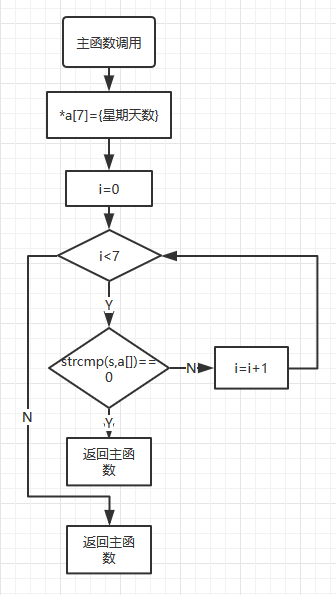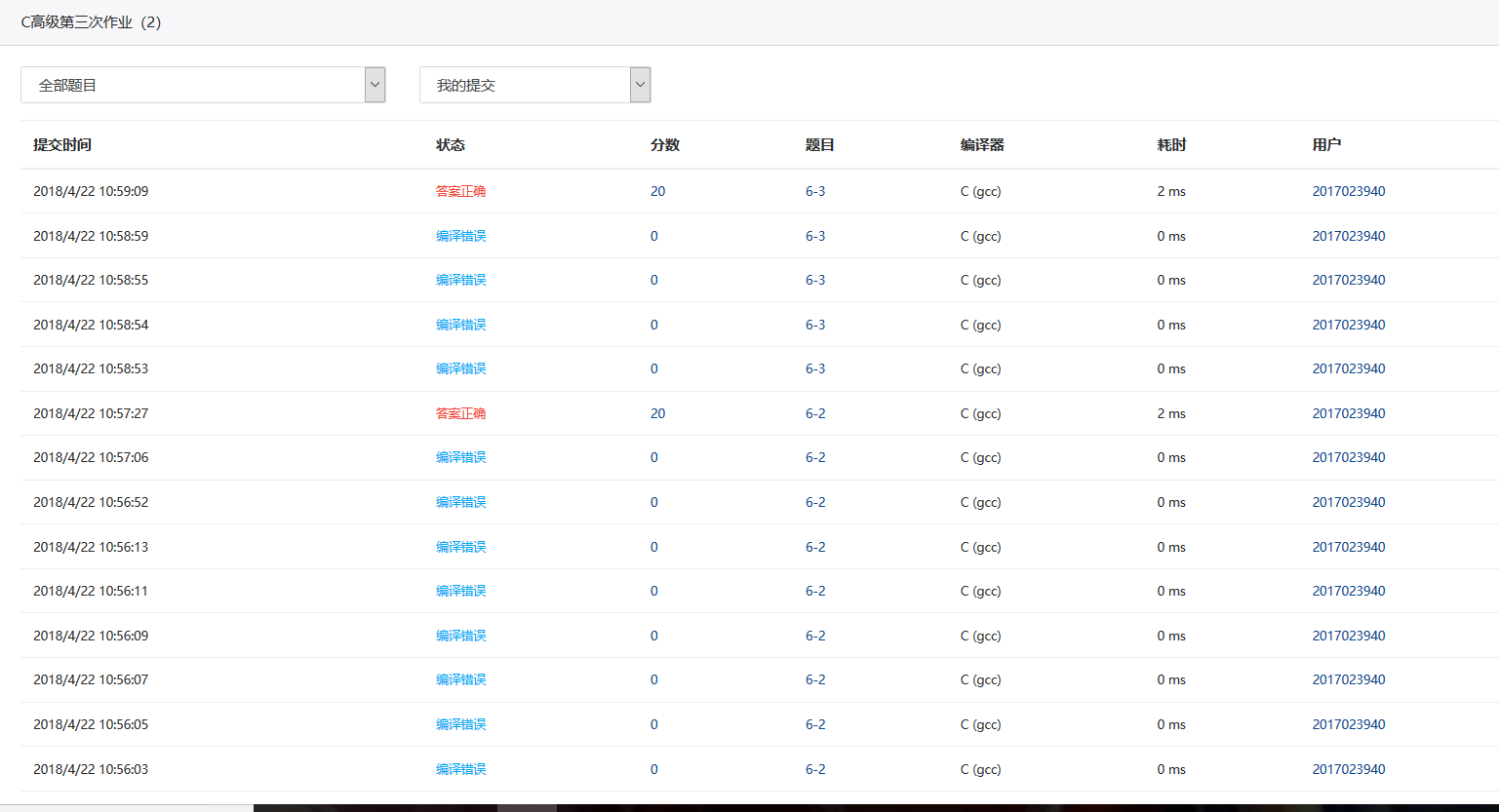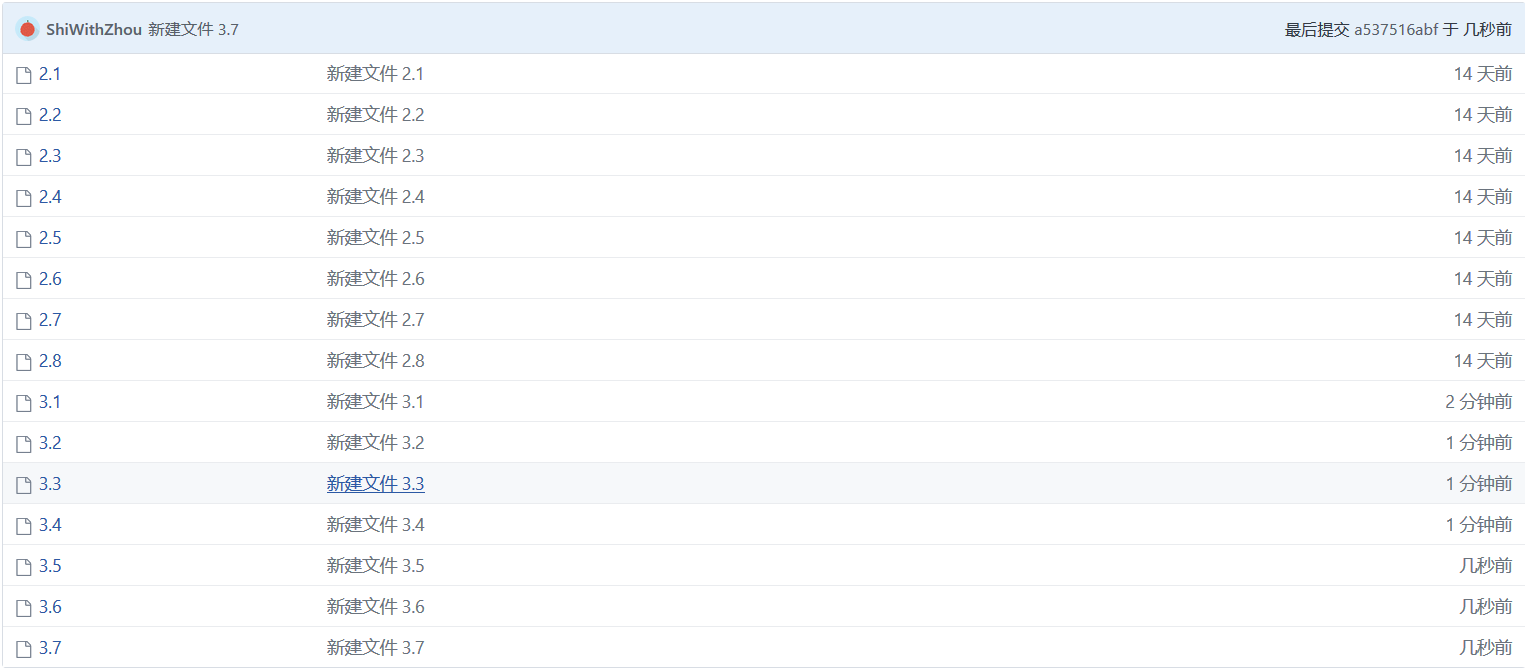# 2018第三次作业。

### 未来两周学习内容

• 指针数组
• 指向指针的指针
• 用指针数组处理多个字符串
• 命令行参数
• 指针作为函数的返回值
• 链表
• 链表的概念
• 链表的常用操作

### 作业要求一 （20分）

1）C高级第三次PTA作业（1）#### 6-1 输出月份英文名

char *getmonth( int n )
{
char *month;
char *mon = {"January","February","March","April","May","June","July","August","September","October","November","December" };
month = NULL;
if(n <= 0 || n > 12)
return month;
month = mon[n - 1];
return month;
}#### 6-2 查找星期

int getindex( char *s )
{
int i;
char *a= { "Sunday","Monday","Tuesday","Wednesday","Thursday","Friday","Saturday" };
for (i = 0; i < 7; i ++)
{
if (strcmp(s, a[i]) == 0)
break;
}
if (i == 7)
i = -1;
return i;
}

#### 6-3 计算最长的字符串长度

int max_len( char *s[], int n )
{
int a[n],i,k,max = 0;
for(i = 0;i < n;i ++)
{
char *b = s[i];
for(k = 0;;k ++)
{
if(b[k] == '\0')
{
a[i] = k;
break;
}
}
if(a[max] < a[i])
max = i;
}
return a[max];
}

#### 6-4 指定位置输出字符串

char *match( char *s, char ch1, char ch2 )
{
int i = 0,k;
for(;s[i] != 0;i ++)
{
if(s[i] == ch1)
{
for(k = i;s[k] != 0;k ++)
{
printf("%c",s[k]);
if(s[k] == ch2)
break;
}
printf("\n");
return s+i;
}
}
printf("\n");
*s = '\0';
return s;
} 

2）一道编程题：

3）C高级第三次PTA作业（2）#### 6-1 奇数值结点链表

struct ListNode *readlist()
{
while(1)
{
p=(struct ListNode*)malloc(sizeof(struct ListNode));
scanf("%d",&p->data);
if(p->data==(-1))
break;
n->next=p;
n=p;
}
n->next=NULL;
}
struct ListNode *getodd( struct ListNode **L )
{
struct ListNode *i,*j,*k,*h1,*h2;
j=h1=(struct ListNode*)malloc(sizeof(struct ListNode));
h1->next=NULL;
k=h2=(struct ListNode*)malloc(sizeof(struct ListNode));
h2->next=NULL;
i=*L;
int n=0;
for(;i;i=i->next)
{
if((i->data)%2==1)
{
j->next=i;
j=i;
}
else
{
k->next=i;
k=i;
}
}
j->next=NULL;
k->next=NULL;
*L=h2->next;
return h1->next;
}

#### 6-2 学生成绩链表处理

struct stud_node *createlist()
{
while(1)
{
p=(struct stud_node*)malloc(sizeof(struct stud_node));
scanf("%d ",&p->num);
if(p->num==0)
break;
scanf("%s %d",&p->name,&p->score);
n->next=p;
n=p;
}
n->next=NULL;
}
struct stud_node *deletelist( struct stud_node *head, int min_score )
{
struct stud_node *p,*k;
p=(struct stud_node*)malloc(sizeof(struct stud_node));
k=p;
{
{
}
}
k->next=NULL;
return p->next;
}

#### 6-3 链表拼接

struct ListNode *mergelists(struct ListNode *list1, struct ListNode *list2)
{
struct ListNode *h,*p,*i,*k;
h=(struct ListNode*)malloc(sizeof(struct ListNode));
p=h;
i=list1;
k=list2;
while(i!=NULL&&k!=NULL)
{
if(i->data<k->data)
{
p->next=i;
i=i->next;
}
else
{
p->next=k;
k=k->next;
}
p=p->next;
}
while(i)
{
p->next=i;
i=i->next;
p=p->next;
}
while(k)
{
p->next=k;
k=k->next;
p=p->next;
}
p->next=NULL;
return h->next;
}

#### 要求三、学习总结和进度（15分）

1、总结两周里所学的知识点，回答下列问题？

（1）如何理解指针数组，它与指针、数组有何关系？为何可以用二级指针对指针数组进行操作？

（2）将C高级第三次PTA作业（1）任何一个题目改为使用二级指针对指针数组进行操作。

（3）用指针数组处理多个字符串有何优势？可以直接输入多个字符串给未初始化的指针数组吗？为什么？

2、将PTA作业的源代码使用git提交到托管平台上，要求给出上传成功截图和你的git地址。3、点评3个同学的本周作业（在作业中给出被点评同学博客的链接），并邀请3名同学点评你的作业，无点评作业(你的作业未被3人点评)/或者没有回复同学或老师的点评都倒扣该题分数。

4、请用表格和折线图呈现你本周（4/9 8:00~4/23 8:00）的代码行数和所用时间、博客字数和所用时间posted @ 2018-04-22 11:11  shilcz  阅读(385)  评论(7编辑  收藏  举报